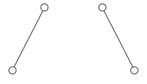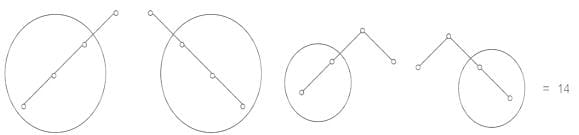Test: Tree

# Test: Tree

Test Description

## 10 Questions MCQ Test Network Theory (Electric Circuits) | Test: Tree

Test: Tree for Electrical Engineering (EE) 2023 is part of Network Theory (Electric Circuits) preparation. The Test: Tree questions and answers have been prepared according to the Electrical Engineering (EE) exam syllabus.The Test: Tree MCQs are made for Electrical Engineering (EE) 2023 Exam. Find important definitions, questions, notes, meanings, examples, exercises, MCQs and online tests for Test: Tree below.
Solutions of Test: Tree questions in English are available as part of our Network Theory (Electric Circuits) for Electrical Engineering (EE) & Test: Tree solutions in Hindi for Network Theory (Electric Circuits) course. Download more important topics, notes, lectures and mock test series for Electrical Engineering (EE) Exam by signing up for free. Attempt Test: Tree | 10 questions in 30 minutes | Mock test for Electrical Engineering (EE) preparation | Free important questions MCQ to study Network Theory (Electric Circuits) for Electrical Engineering (EE) Exam | Download free PDF with solutions
 1 Crore+ students have signed up on EduRev. Have you?
Test: Tree - Question 1

### Which of the following is not true for tree and graph?

Detailed Solution for Test: Tree - Question 1

Tree:

A tree is a connected subgraph of a connected graph containing all the nodes of the graph but containing no loops, i.e., there is a unique path between every pair of nodes.

The number of closed paths in a tree of the graph is zero. Therefore is not true for tree and graph.

Twig: The branches of the tree are called twigs.

Link: Those branches of the graph which are not in the tree.

Co-tree: All the links of a tree together constitute complement of the tree and is called co-tree, in which the number of branches is equal to b - (n - 1)

Where b is the number of branches of the graph.

Number of twigs: t = n - 1

Number of links: L = b - t = b – n + 1

Test: Tree - Question 2

### Which one of the following property is correct for a red-black tree?

Detailed Solution for Test: Tree - Question 2

A red-black tree is a balanced binary search tree with the following properties:

• Every node is colored red or black.
• Every leaf is a NIL node and is colored black.
• If a node is red, then both its children are black.
• Every simple path from a node to a descendant leaf contains the same number of black nodes.
Test: Tree - Question 3

### The degree of a tree is the _____ degree of a node in the tree.

Detailed Solution for Test: Tree - Question 3

Concept:

Tree:

• A tree consists of nodes or vertices that store information and often are labeled by a number or a letter.
• A tree consists of directed edges or undirected edges or both.
• The number of subtrees of a node is called its degree.
• A leaf or a terminal node is a node of degree zero
• A node that is not a leaf is called an interior node or an internal node

The degree of a node is the number of its children. The degree of a tree is the maximum degree of any of its nodes.

Test: Tree - Question 4

The maximum number of binary trees that can be formed with 4 unlabeled nodes is

Detailed Solution for Test: Tree - Question 4

# unlabelled binary trees with 1 node

= 1

# with 2 nodes : -= 2

# with 3 nodes :-

# with 4 nodes :-Test: Tree - Question 5

An undirected graph G which is connected and acyclic is called ____________

Detailed Solution for Test: Tree - Question 5

An undirected graph G which is connected and acyclic is termed as a tree. G contains no cycles and if any edge is added to G a simple cycle is formed.

Test: Tree - Question 6

What is a star tree?

Detailed Solution for Test: Tree - Question 6

A star tree of order n is a tree with as many leaves as possible or in other words a star tree is a tree that consists of a single internal vertex and n-1 leaves. However, an internal vertex is a vertex of degree at least 2.

Test: Tree - Question 7

The tree elements are called __________

Detailed Solution for Test: Tree - Question 7

Every tree element is called a node and the lines connecting the elements are called branches. A finite tree structure has a member that has no superior and is called the “root” Or root node. Nodes that have no child are called leaf nodes.

Test: Tree - Question 8

What is a bipartite graph?

Detailed Solution for Test: Tree - Question 8

A graph is called a bipartite graph if and only if it contains no cycle of odd length. Every tree is a bipartite graph and a median graph.

Test: Tree - Question 9

Two labeled trees are isomorphic if ____________

Detailed Solution for Test: Tree - Question 9

The number of labeled trees of k number of vertices is kn-2. Two labeled trees are isomorphic if their graphs are isomorphic and the corresponding points of the two trees have the same labels.

Test: Tree - Question 10

A polytree is called _______

Detailed Solution for Test: Tree - Question 10

A directed acyclic graph is known as a polytree whose underlying undirected graph is a tree. In other words, a directed tree is a directed graph which would be tree if the directions on the edges were ignored.

## Network Theory (Electric Circuits)

23 videos|63 docs|60 tests
 Use Code STAYHOME200 and get INR 200 additional OFF Use Coupon Code